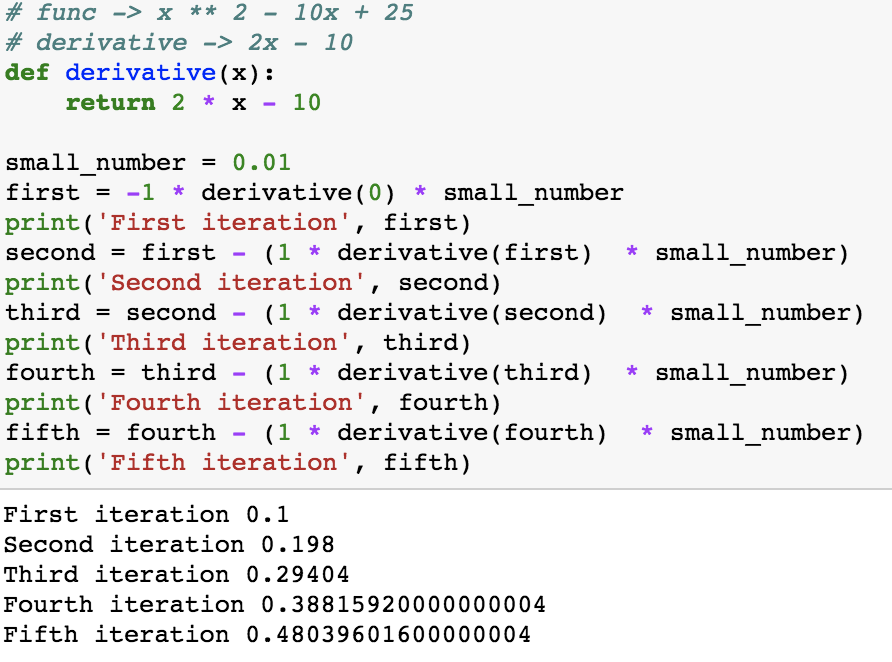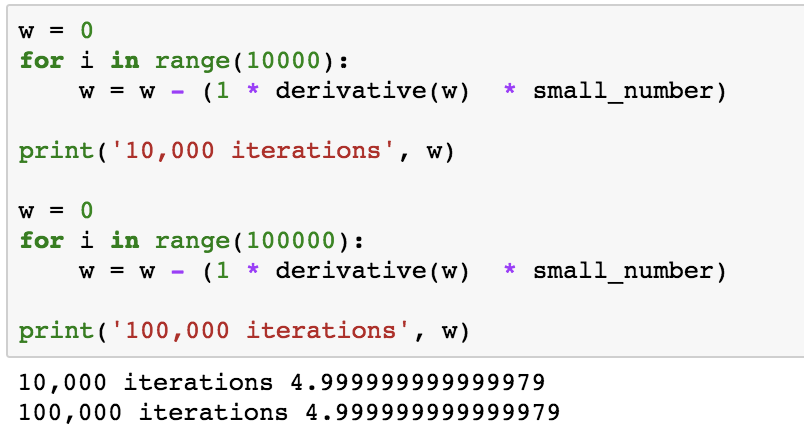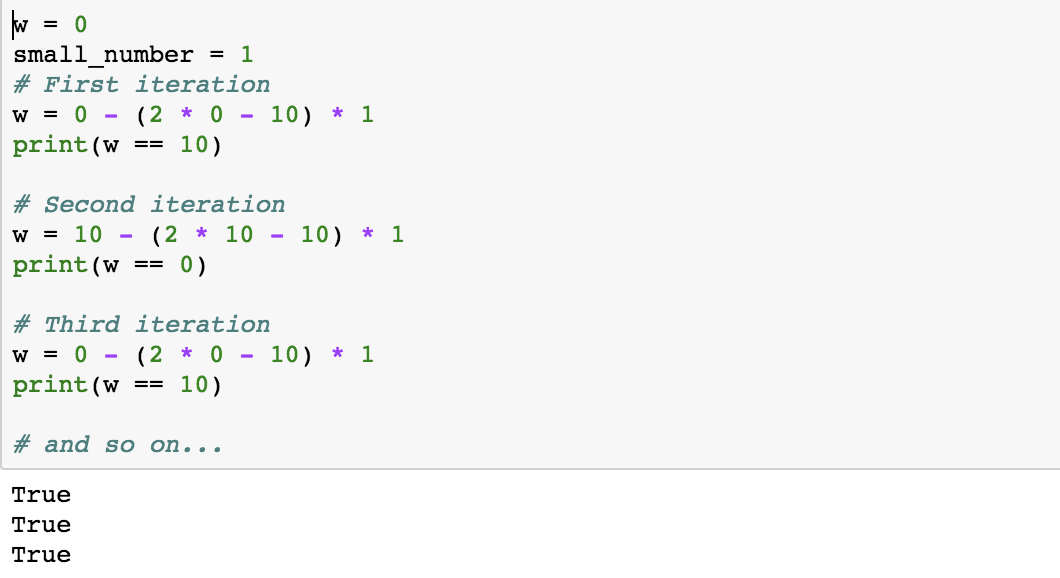Ben Brostoff

# 09 Oct 2017 Gradient Descent Intuition (ML Series, Part 2)

How do machines learn?

One answer is by getting fewer answers wrong over time. One technique for decreasing wrongness is gradient descent.

In this post, I want to explore gradient descent as a standalone topic. In the next post in this series, I’ll discuss its relationship with machine learning, and run through a basic example of why gradient descent is useful in helping machines get fewer answers wrong.

Gradient Descent - Find The Minimum

Matt Nedrich over at Atomic Object has an excellent definition of gradient descent:

At a theoretical level, gradient descent is an algorithm that minimizes functions. Given a function defined by a set of parameters, gradient descent starts with an initial set of parameter values and iteratively moves toward a set of parameter values that minimize the function. This iterative minimization is achieved using calculus, taking steps in the negative direction of the function gradient.

Gradient descent can be thought of as a synonym for slope. Since slope is rise / run, and a horizontal line has a slope of 0 (0 rise over infinite run), finding the slope at the function’s minimum can be thought of as “descending” to a gradient of zero. The name gradient descent makes sense, because once the gradient decent algorithm has run for a given function, the computed parameters will descend the slope at the computed point to zero.

Providing clear examples of the how of ML are among the reasons I’m writing this blog series, so let’s use gradient descent on the following function:

`(x - 5) ** 2`

I write this function in this form as opposed to `x ** 2 - 10x + 25` because it’s easy to see that the function is minimized at 5. Gradient descent should return `5` for `x` if the algorithm lives up to its name.

The gradient descent algorithm itself is all of one line, but importantly requires 1) the derivative (also synonymous with slope) of the function and 2) a small number to descend the gradient. The small number - which we’ll set to `0.01` in this example - is necessary because it allows the gradient to move on each iteration of the algorithm. The derivative can be computed through the power rule, which I had to review via the provided link from Khan academy. In short, moving the exponent `2` down and raising the result to `2-1` returns a derivative function of `2(x-5) ** 1` or `2x - 10`. We now have the parameters necessary to run gradient descent for a few iterations:What this algorithm does is 1) take the previous minimized value (or start at zero) and 2) subtract the slope function with the previous minimum value passed in, multiplied by the small number.

And if run for 10,000 iterations or even 100,000 iterations, with `0.01` as the small number, `x` converges to `5`.But what if instead of a small number we used a huge number? What is clear from experimentation is that numbers larger than one will never allow the solution to converge to `5`. While a small number of `0.99` converges to `5`, a small number of `1.0` bounces the solution back and forth between `10` and `0` forever. A couple of iterations in this sequence are instructive:The value selected for the small number is extremely important. Selection of the small number is an example of hyperparamater tuning in machine learning, which we’ll explore in a later post.

Please note all the code for this post can be found on my GitHub.

Why Does Gradient Descent Help Machines Learn?

The small number mentioned above is called the learning rate in ML contexts, and drives the learning in machine learning. The function the gradient descent algorithm is applied on is the loss function, where loss is the difference between the prediction made from a machine learning example and actual reality. For example, if an ML model predicted an NFL running back will finish with `17` touch downs for the 2016 season and the reality is `11`, the loss in this example is `6`.

In the next post, I’m excited to explore an actual application of gradient descent in machine learning.